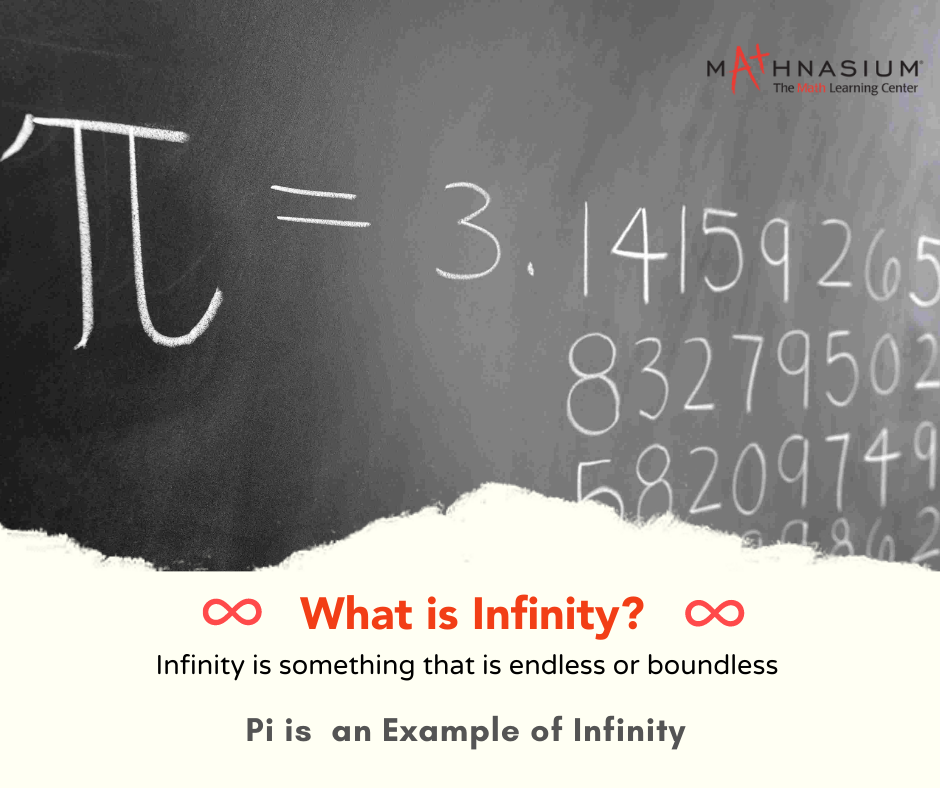877-601-6284
Get Started by Finding a Local Center

# What is Infinity?

Jun 15, 2022 | Location West Palm BeachHave you ever been in the playground playing a game of naming the biggest number you can think of? How quickly does someone say “infinity?” Then the next person says, “infinity plus one,” and the game never seems to stop. But is infinity plus one really bigger than infinity?

Georg Cantor (1845–1918) was one of the first mathematicians who studied infinity. So what is infinity, exactly? In Math, “infinity” is the concept describing something larger than the natural number. It generally refers to something without any limit. It is a state of endlessness or having no time, space, or other quantity limits.

The common symbol for infinity, ∞, was invented by the English mathematician John Wallis in 1657. The infinity symbol, called the lemniscate, is used more often to represent a potential infinity, rather than to represent an actually infinite quantity. Actual infinite quantities would be things such as ordinal numbers and cardinal numbers.

Is Infinity a Number?

So, is infinity a number? Not quite.

Infinity is best described as a concept or an idea, rather than a concrete number.

In math, infinity is often treated as a number in that it can be used to count or measure things, but it is not considered a natural or a real number. Nothing is bigger than infinity, and infinity is neither odd nor even.

The direct use of the infinity symbol in math arises in order to compare the sizes of the sets such as the set of counting numbers, the set of points in the real number, and so on.

Properties

Here are some important properties to remember when working with infinity!

If any number is added to infinity, the sum is also equal to infinity.

∞ + ∞ = ∞

-∞ + -∞ = -∞

Subtraction Property:

Subtracting infinity from infinity will result in an indeterminate form. (The term “indeterminate” means an unknown value or anything that can't be defined)

``   ∞ - ∞ = Indeterminate form``

Multiplication Property :

If a number is multiplied by infinity, then the value of the product is also equal to infinity.

∞ × ∞ = ∞

-∞ × ∞ = -∞

-∞ × -∞ = ∞

Some Special Properties:

If x is any integer, then;

x + (-∞) = -∞

x + ∞ = ∞

x – (-∞) = ∞

x – ∞ = -∞

For x > 0:

x × (-∞) = -∞

x × ∞ = ∞

For x < 0:

x × (-∞) = ∞

x × ∞ = -∞

Value of Infinity

A mathematical infinity is the conceptual representation of such a numberless number. In many instances, it is treated as if it were a number that counts or measures: "an infinite number of terms, but it is not the same sort of number as a natural number.

However, infinity is the conceptual expression of such a numberless number in math. It is often treated as if it were a number that counts or measures things: “an infinite number of terms, but it is not the same sort of number as natural or real numbers.

For example, A list of natural numbers 1, 2, 3, 4,…….no matter how long you count for, it can never reach the end of all numbers. Similarly, in the never-ending universe, if you travel in the fastest spaceship, you can’t reach the end of an unending universe.

Examples

Below you can find some fun facts about some mathematical concepts that stretch infinitely!

The sequence of natural numbers is infinite. {1, 2, 3, …}

A-line or even a line segment consists of infinite points.

Similarly, a circle consists of infinite points.

The number pi (π) goes on forever. (3.14159…)

Certain fractions are finite, but they are infinite when written as decimal numbers. (1/3 is 0.333…)

The number of prime numbers is infinite.

The number phi (Φ) is the golden ratio, (1 + √5)/2, which is an infinite decimal number 1.618…

While astronomers can see the edge of the Universe formed by the Big Bang, it’s unknown whether it will expand forever (infinitely) or stop and contract again (finite).

Fractals are structures that can be magnified infinitely without losing their structure.

In complex number theory, dividing 1 by 0 is an infinity that doesn’t collapse. (On a calculator, dividing any number by Zero is just an error code.)

There are many examples of infinite series in math. For example, 1 + 1/2 + 1/3 + … is an infinite series.

Infinity is a means to describe anything that is endless, making it impossible to measure. It refers to unending time, a series of numbers that continues forever, or a perpetual series of operations.. Most things we know have an end, but infinity does not.

##### Locations near
me
Capitol Hill DC 28.48 mi
621 Pennsylvania Ave SE
1st-floor unit
Washington, DC 20003